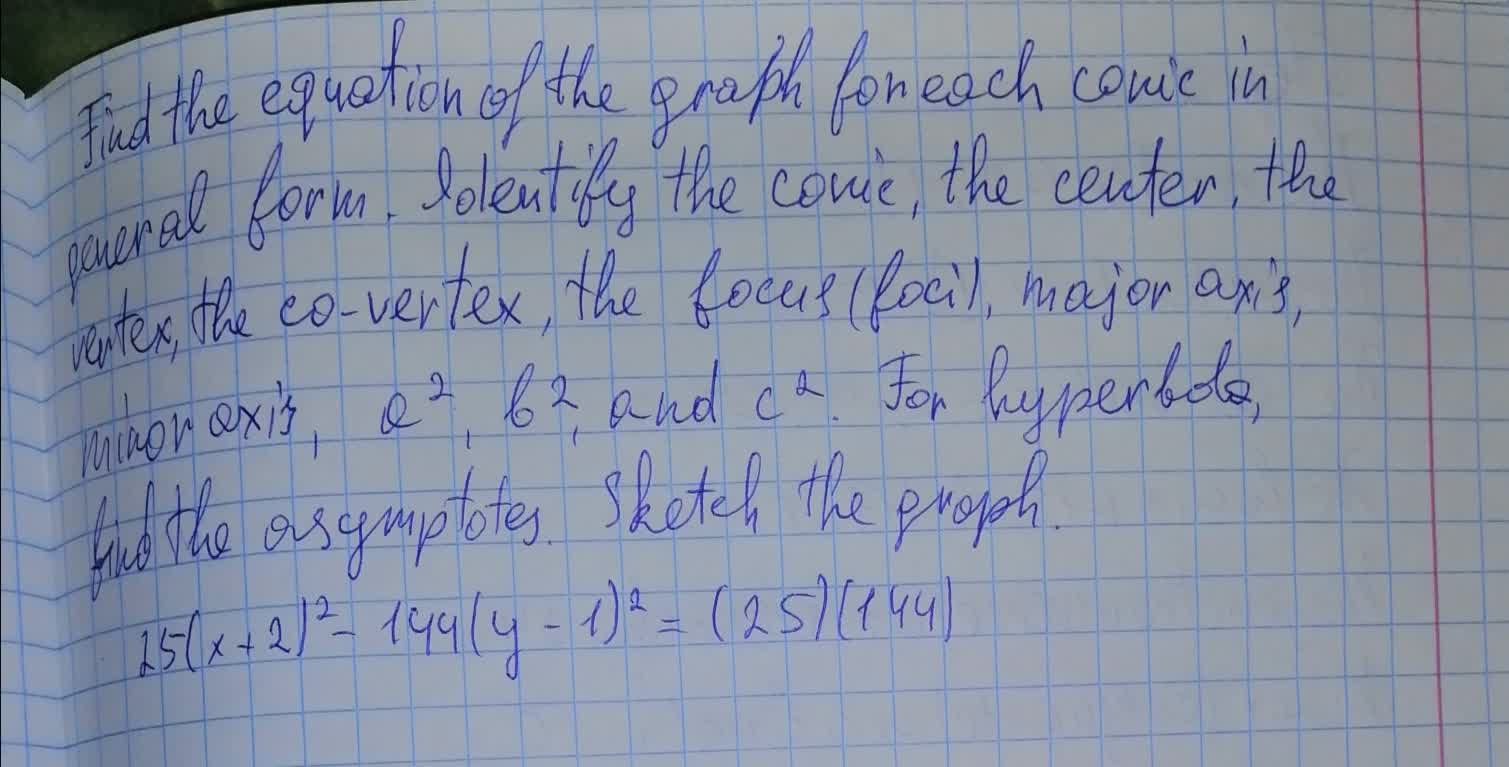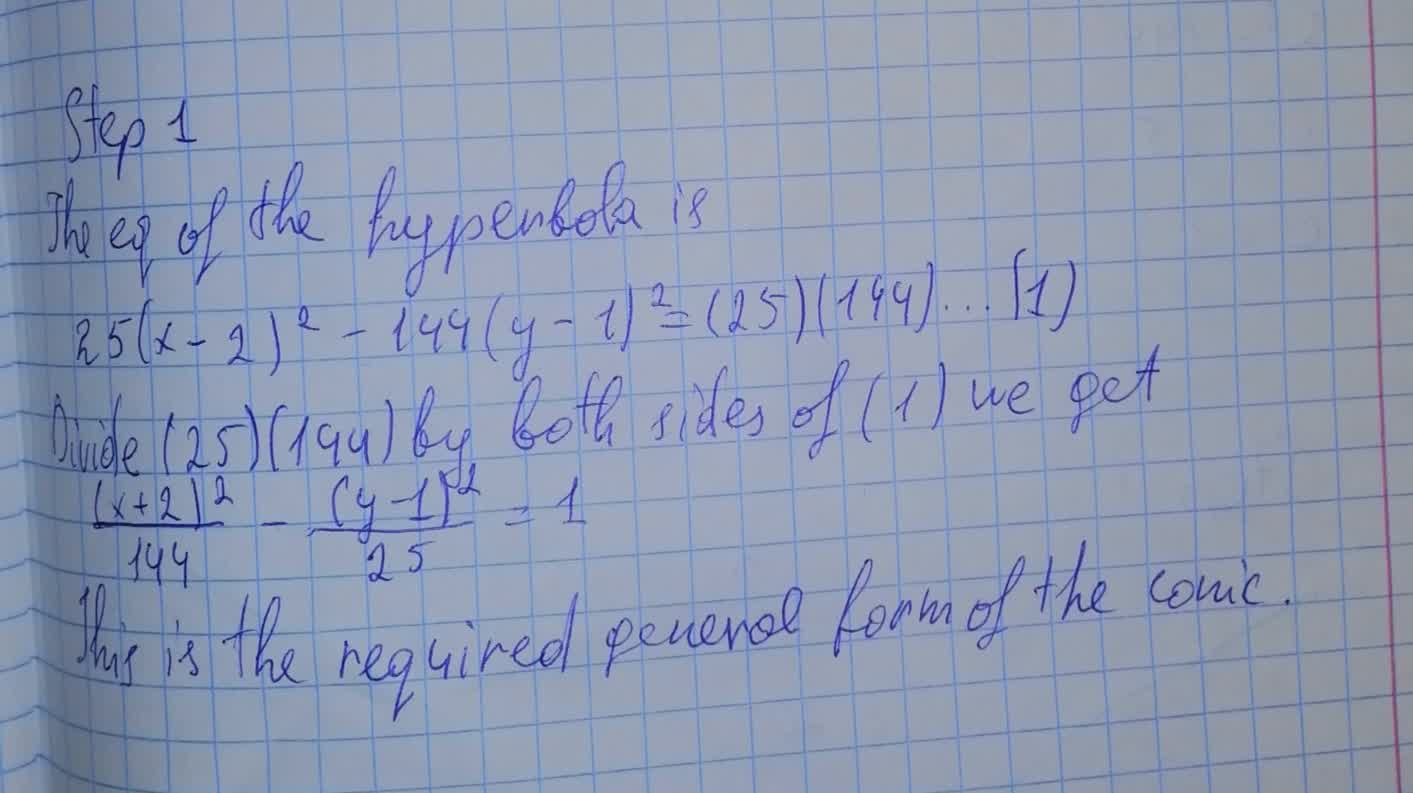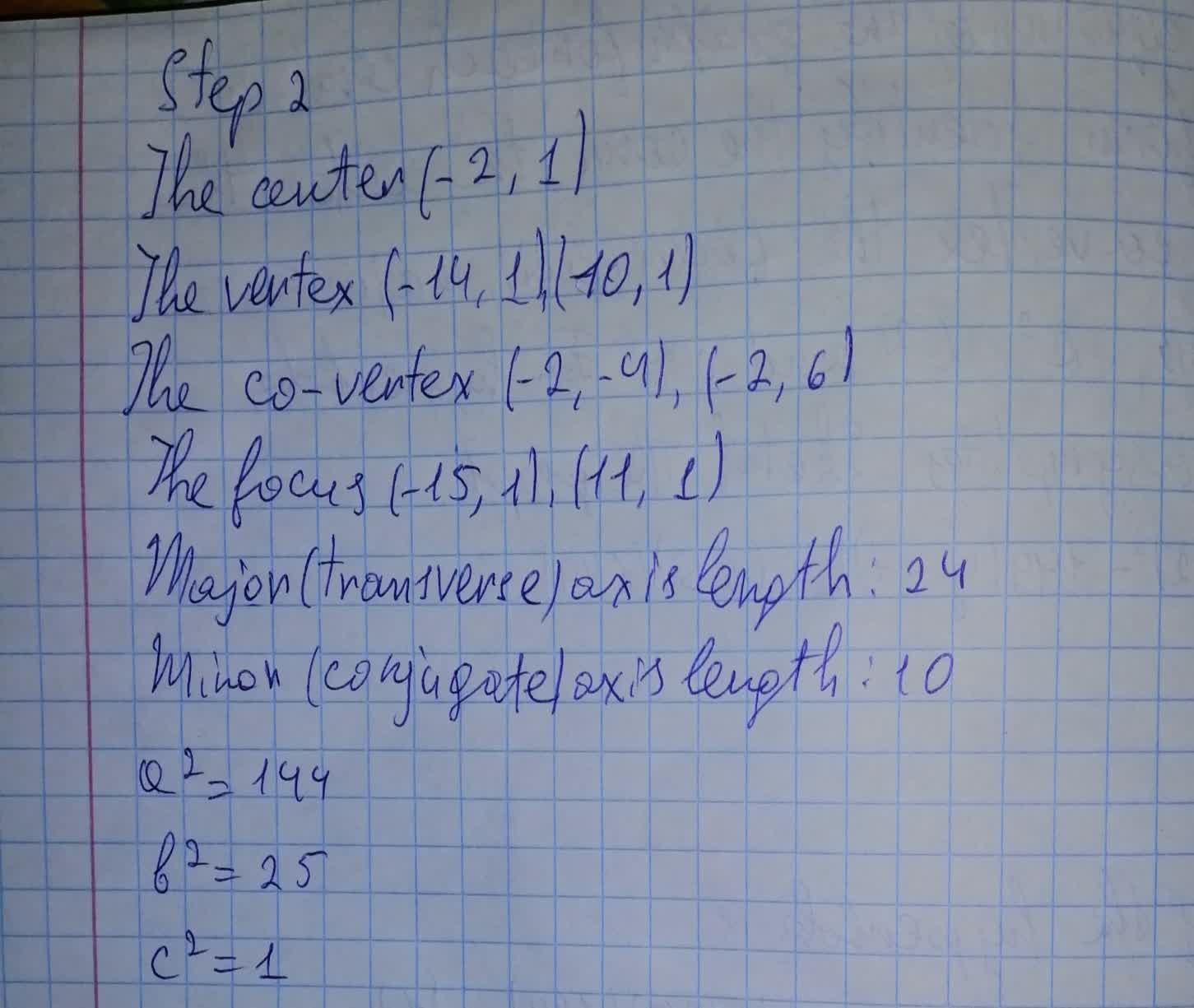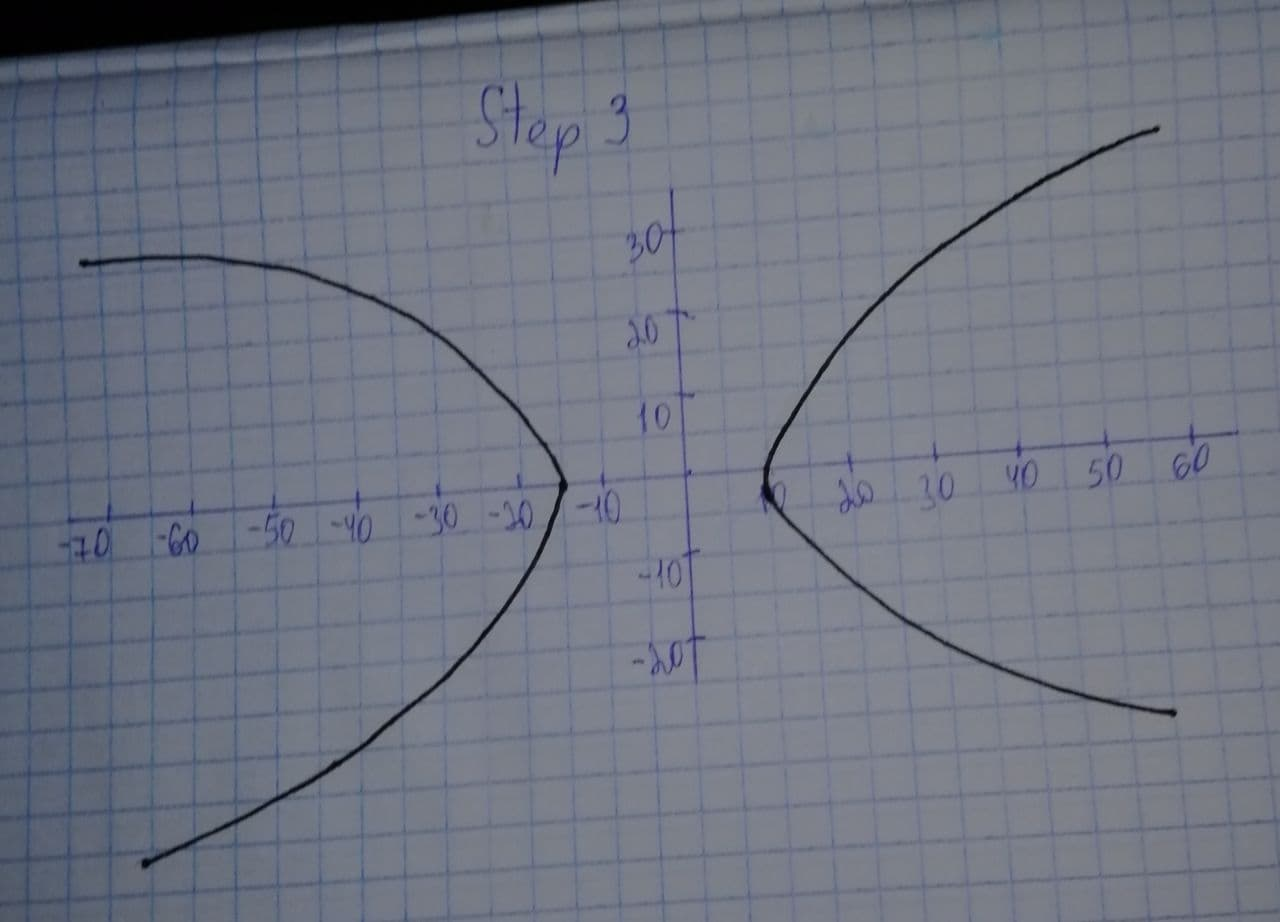Find the equation of the graph for each conic in general form. For hyperbola , find the asymptotes. Sketch the graph.Mylo O'Moore 2021-08-07 Answered
Find the equation of the graph for each conic in general form. Identify the conic , the center , the vertex , the co-vertex , the focus(foci) , major axis , minor axis , $$\displaystyle{a}^{{2}}$$ , $$\displaystyle{b}^{{2}}$$ and $$\displaystyle{c}^{{2}}$$. For hyperbola , find the asymptotes. Sketch the graph.
$$\displaystyle{25}{\left({x}+{2}\right)}^{{2}}-{144}{\left({y}-{1}\right)}^{{2}}={\left({25}\right)}{\left({144}\right)}$$• Questions are typically answered in as fast as 30 minutes

Solve your problem for the price of one coffee

• Math expert for every subject
• Pay only if we can solve itlamusesamuset